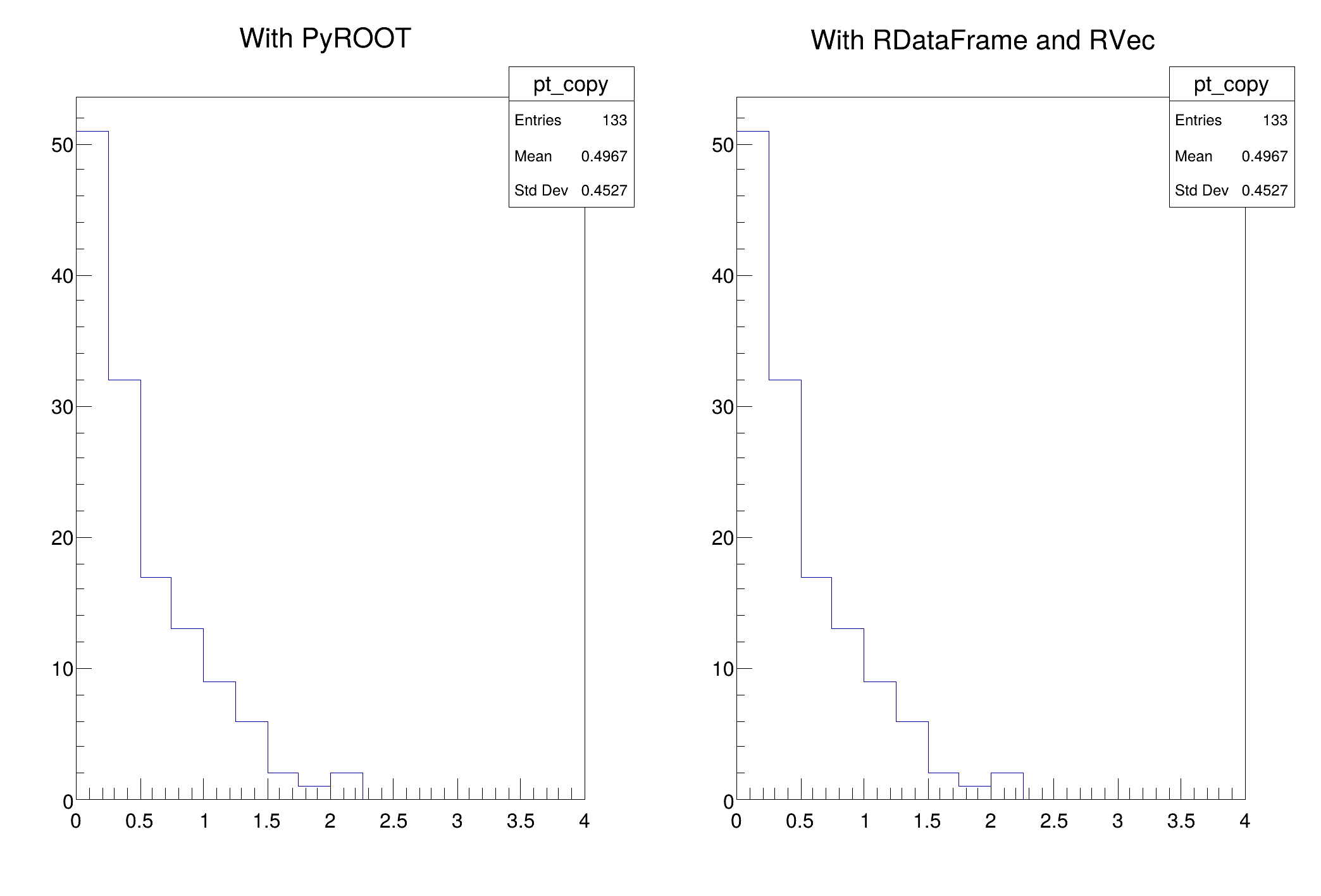ROOT   Reference Guidedf017_vecOpsHEP.py File Reference

df017_vecOpsHEP

## Detailed DescriptionUse RVecs to plot the transverse momentum of selected particles.

This tutorial shows how VecOps can be used to slim down the programming model typically adopted in HEP for analysis.

import ROOT
filename = ROOT.gROOT.GetTutorialDir().Data() + "/dataframe/df017_vecOpsHEP.root"
treename = "myDataset"
def WithPyROOT(filename):
from math import sqrt
f = ROOT.TFile(filename)
h = ROOT.TH1F("pt", "With PyROOT", 16, 0, 4)
for event in f.myDataset:
for E, px, py in zip(event.E, event.px, event.py):
if (E > 100):
h.Fill(sqrt(px*px + py*py))
h.DrawCopy()
def WithRDataFrameVecOpsJit(treename, filename):
f = ROOT.RDataFrame(treename, filename)
h = f.Define("good_pt", "sqrt(px*px + py*py)[E>100]")\
.Histo1D(("pt", "With RDataFrame and RVec", 16, 0, 4), "good_pt")
h.DrawCopy()
## We plot twice the same quantity, the key is to look into the implementation
## of the functions above
c = ROOT.TCanvas()
c.Divide(2,1)
c.cd(1)
WithPyROOT(filename)
c.cd(2)
WithRDataFrameVecOpsJit(treename, filename)
c.SaveAs("df017_vecOpsHEP.png")
print("Saved figure to df017_vecOpsHEP.png")Date
March 2018

Definition in file df017_vecOpsHEP.py.

ROOT::RDataFrame
ROOT's RDataFrame offers a high level interface for analyses of data stored in TTree,...
Definition: RDataFrame.hxx:42
sqrt
double sqrt(double)